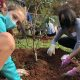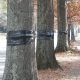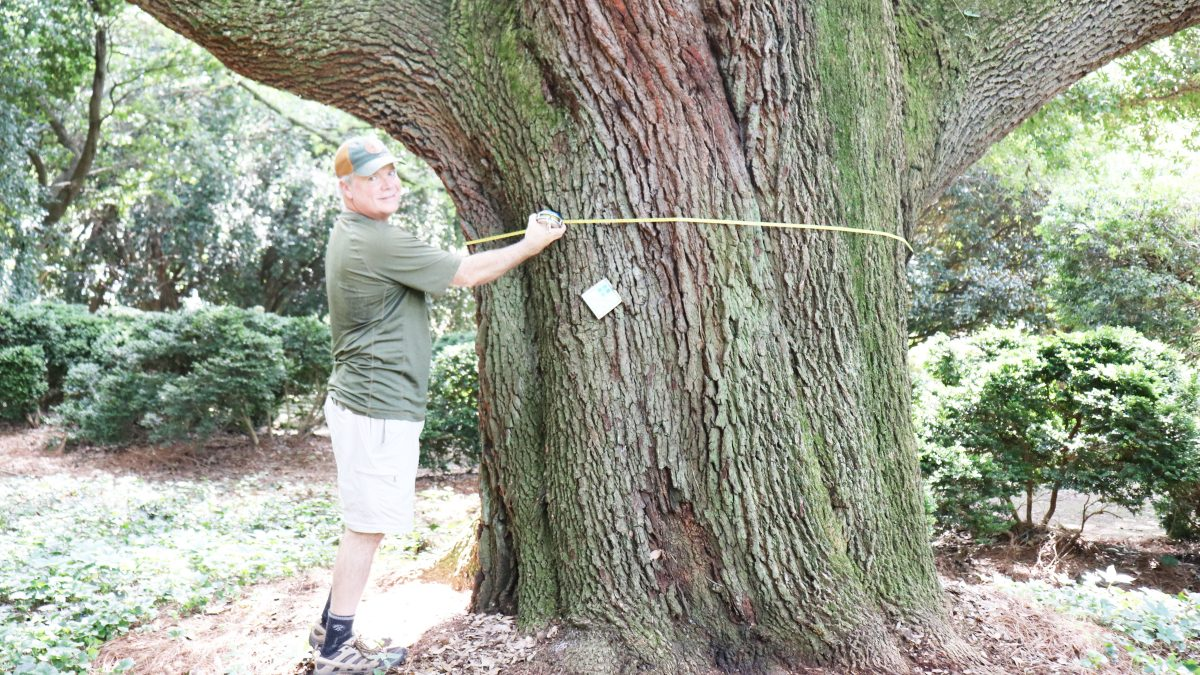﻿ Tree Age Equation - TreesCharlotte

# Tree Age Equation###### A record-setting season!
11.24.20###### Cankerworm Banding
11.30.20Did you know that you can determine the approximate age of any tree just by using some measuring tape and simple math? Follow these steps and see for yourself! 🌳✖️❤️

1. Measure the circumference (in inches) of the tree’s trunk at breast height (or 4.5 feet above the ground). This is called the CBH.

2. Take the CBH that you measured and divide it by pi (or 3.14159). This will give you the DBH (diameter at breast height) of the tree.

3. Multiply the DBH by the “Growth Factor” that corresponds to the species of tree that you’re working on (found in the table below).

4. You’re done! That number is the approximate age of the tree! 🌳

Here’s the complete equation and an example:

CBH / pi = DBH

DBH x Growth Factor = Age

So, if I have a Sugar Maple with a 12 inch circumference…

12 / 3.14159 = 3.8197

3.8197 x 5.0 = 19

My Sugar Maple is approximately 19 years old!

Looking for the Urban Forest Master Plan?